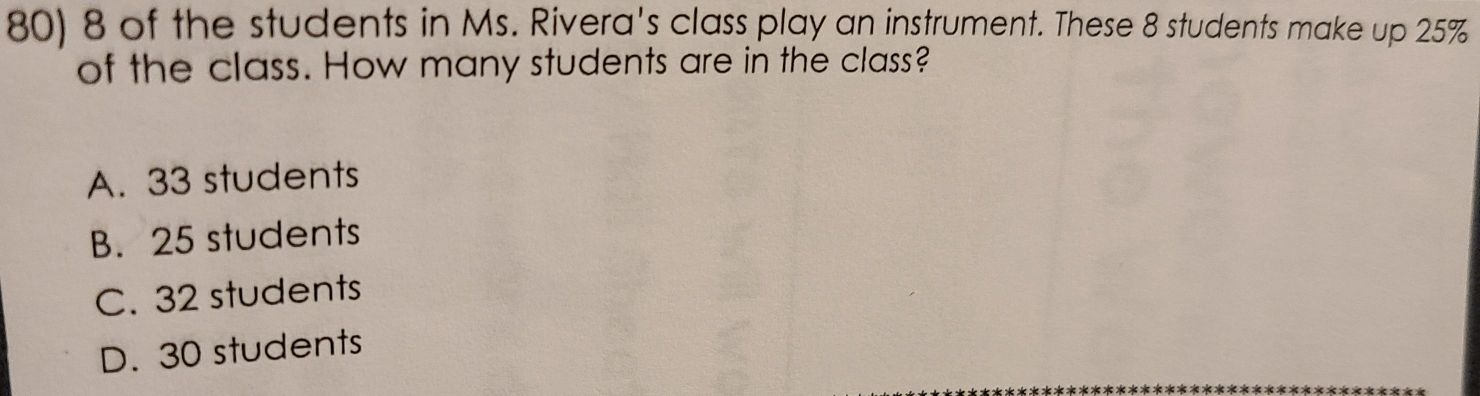### Still have math questions?

Arithmetic
Question80) $$8$$ of the students in Ms. Rivera's class play an instrument. These $$8$$ students make up $$25 \%$$ of the class. How many students are in the class?

A. $$33$$ students B. $$25$$ students C. $$32$$ students D. $$30$$ students